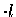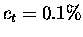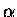Next: TOF series B Up: Yield measurements Previous: TOFY: Time-of-flight Yield

### TOF series A

The measurement for a 3 Tlayer in Series A () had an additional difficulty in the background subtraction, which was overcome by using different methods of background estimation and comparing them.

The most suitable background run for theseries (BG6 in Table 8.2, Page) had rather limited statistics with about 1/9 of GMUs of the production run. Performing our standard background subtraction procedure using BG6, illustrated in Fig. 8.9, exhibited two potential systematic effects: (a) a Si1 to Si2 detector ratio of up to about 30% was observed in the yield using BG6 for 3 T(see values with in Table 8.6), which appear to be due mostly to asymmetry in the background BG6, not the signal, and (b) there appears to be low energy tails in the background subtracted spectra down to 2 MeV (2000 ch) (see Fig. 8.9, and Table 8.6).

Using instead BG5, which does not have DS 500 TH2 under the DS deuterium layer, makes the Si1/Si2 ratio consistent with 1. However, the lack of the H2 layer in BG5 could cause an underestimate of the background, since muon decay electrons are a major source of background especially at lower energies. In fact, yields with BG5 are higher than those with BG6 in all cases, but clearly with a lower energy cut (see values with in Table 8.6).

Another possible run to be used for background subtraction is BG7, which had an emission target with ct=0.2%, and no H2 DS substrate layer. Despite the difference in ct, which might possibly introduce further systematic error, this run had much better statistics (about 3 times that of BG6).

Given this situation for the 3 Tmeasurement in theseries, we made several different background subtractions, and compared them to see the systematic effects. Method 1 is the standard procedure using BG6, but with a wide energy cut ( 2.5 < E < 3.7 MeV) to account for the possibility that the lower energy tails are due indeed to realparticle events, which gave the Si averaged yield of /GMU.

The energy spectra obtained with Method 1 had nonzero counts even at energies much higher than the fusion signal, where we expect an average of counts consistent with zero. In Method 1-b, taking into account the possibility that this is due to a potential error in the relative normalization (although the ADC blocking effect in Si detectors was corrected carefully in Section 8.1.2), we fitted the region between 4000 and 8000 ch with a constant base line, and subtracted that from the yield from Method 1 above to obtain . Using a line with a slope or exponential curve in the fit gave a larger base line yield (hence smaller fusion yield), but we use the value Ymeth1-b as our upper limit in calculating the average later.

Method 2 used the entire background data set available (sum of BG5, BG6, and BG7) to make a first order background subtraction (see bottom in Fig. 8.10). But keeping in mind some differences in the conditions, we made an additional, second order correction from the above background. From the narrowly defined signal region (denoted SG, 3.1 <E< 3.7 MeV), the average of counts from the region below (shown as B-LO, 2.2 <E<2.8 MeV) and above (B-HI, 3.8<E<4.6 MeV) were subtracted, i.e., Ymeth2 =SG - 0.5(B-LO + B-HI). With SG= , B-LO= , and B-HI= , we have .

The systematics in Method 2 were checked for the manner in which the additional correction was made; the background cut, B-LO, might contain the signal, which, together with the narrow cut for SG, might lead to over-subtraction of background. We present two more systematic checks of Method 2, in which the second order additional correction was done in a different way, while keeping the first order the same as Method 2.

Method 2-b is simply to use a wider signal region of 2.7 <E< 3.7 MeV, with B-L0 2.1 <E<2.6 MeV and B-HI 3.8 <E<4.3 MeV. Note that because of the low energy structure of background, we do not desire to use the spectrum much lower than 2 MeV. Thus B-LO and B-HI now each have half the energy width of SG. The detector averaged yield thus obtained with Method 2-b is: .

In Method 2-c, illustrated in Fig. 8.11, we have fitted the residual background obtained in Fig. 8.10 with an exponential function. The fit was performed simultaneously in the regions B-LO ( 2.0 <E<2.4 MeV) and B-HI ( 3.8 <E<6.0 MeV), and are plotted with a solid line in Fig. 8.11. The yield was determined in the SG region ( 2.5 < E< 3.7 MeV), which was excluded from the fit, by subtracting the area under the fitted curve. We obtain , where the error is due to the statistics in the SG counts.

Table 8.7: Summary of the detector averaged Si yield for 3 TTOF measurement () with different background subtraction methods. The energy cut dependent corrections (cut efficiency and dd proton contribution) are made. Taking the average of two bold values we obtain /GMU, without the time cut and the nitrogen contamination corrections (see text for the details).
 Method 1 1-b 2 2-b 2-c BG run BG6 BG6 BG5+6+7 BG5+6+7 BG5+6+7 SG cut (MeV) 2.5; 3.7 2.5; 3.7 3.1; 3.7 2.7; 3.7 2.5; 3.7 SG yield (10-6/GMU) 4.43(26) 4.43(26) 4.05(11) 4.64(14) 4.81(15) B-LO cut (MeV) - - 2.2; 2.8 2.1; 2.6 2.0; 2.4 B-HI cut (MeV) - 4.0; 8.0 3.8; 4.4 3.8; 4.3 3.8; 6.0 Fit/sum for ith bin - yi= const. yi=ae-xi/b B yield (10-6/GMU) - 0.38(6) 0.54(6) 0.99(11) 1.36 TOF yield (10-6/GMU) 4.43(26) 4.06(27) 3.52(12) 3.65(18) 3.45(15) dd proton 2.4(9)% 2.4(9)% 0 2.4(9)% 2.4(9)% E cut efficiency 1 1 0.980(3) 1 1 TOFcorr (10-6/GMU) 4.32(26) 3.96(27) 3.59(12) 3.56(18) 3.37(15)

The Si yield for 3 Tin TOF Series A (), using different background subtraction methods, are summarized in Table 8.7. Energy cut width dependent corrections, i.e., the energy cut efficiency and the dd proton contribution, which will be discussed in detail in Sections 8.2.4 and 8.2.3, are applied here.

As can be seen, Method 2, 2-b, 2-c, which all used combined background BG5+6+7 but had different methods of residual background subtraction, gave consistent results, giving some confidence at least in the residual background subtraction procedure. In comparison with Method 1, Method 1-b appears more reliable since it takes into account the possible relative normalization error by subtracting a constant base line. Note that in Series B, the constant term was consistent with zero, as expected. Discarding Method 1, we chose the two extreme values in (Method 1-b and Method 2-c) as representative of the deviations due to the background subtraction procedure. Since these measurements are not completely independent, determining the average and its error is somewhat tricky. Obviously, since the two values are incompatible within in the quoted error, simply taking a weighted mean and combining the error would underestimate the total uncertainty.

Here we adopt a procedure used by the Particle Data Group [229,230], which scales the error bar to give . In our case, with the numbers of points and the free parameter being 2 and 1 respectively (hence dof=1), we scale the error by a factor 1.9 and obtain /GMU.

As for other TOF series A measurements (with), the statistics of the production runs and the standard background run (BG6) are comparable, and the accuracy is not limited by the background statistics. Also because of a better signal-to-background ratio, effects of the background are not as serious. Furthermore, the Si1/Si2 asymmetry in Si yields are at the 10% level for the most part. Thus, we shall use BG6 for our background subtraction. Using other backgrounds in fact gives consistent results for a reasonable range of the energy cuts (Table 8.6).Next: TOF series B Up: Yield measurements Previous: TOFY: Time-of-flight Yield